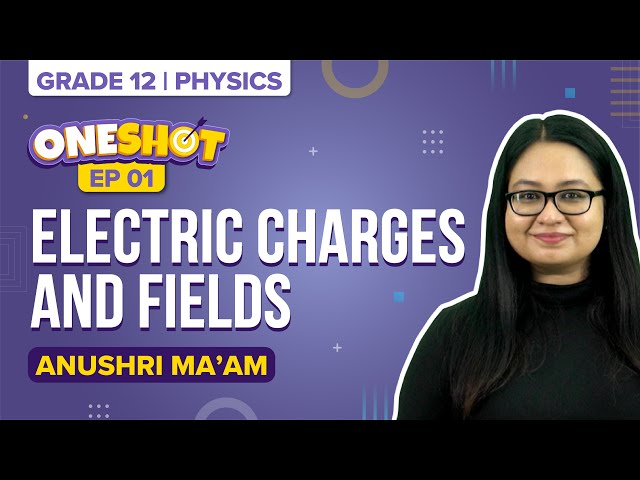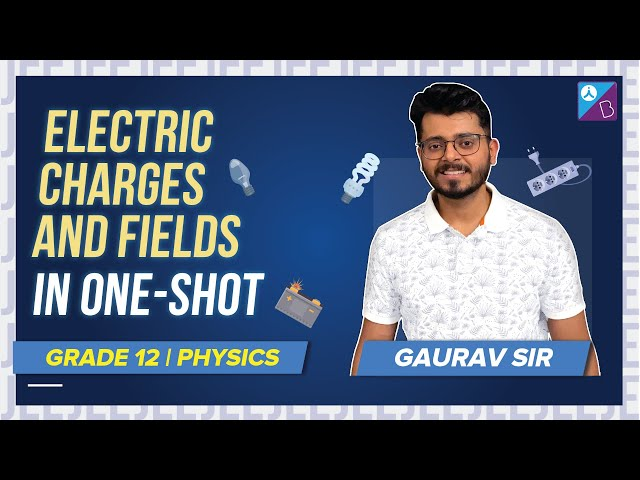# Important Questions for Class 12 Physics Chapter 1 - Electric Charges and Fields

Electric charge is a property that causes particles to experience a force when placed in an electromagnetic field. This force can repel other particles depending on whether they have the same or opposite charges. Positive charges are carried by protons, while negative charges are carried by electrons. An object that does not have a net charge is considered neutral. Classical electrodynamics is applicable to many situations that involve quantum mechanics. Electric charge is a quantity that is conserved, meaning it cannot be created or destroyed. In an isolated system, the total amount of positive charge must always equal the total amount of negative charge. Electric charge is carried by subatomic particles, such as protons and electrons. In most substances, negatively charged particles (electrons) are found outside the positively charged nuclei (made up of protons). If there are more electrons than protons in a piece of matter, it will have a net negative charge. If there are fewer electrons than protons, the substance will have a net positive charge. If the number of electrons and protons is the same, the substance will be neutral.

An electric field is a force field created by electric charges or changing electric currents. It influences the behaviour of charged particles within its area of influence, attracting or repelling them. The electric field is closely related to the magnetic field, as they are both parts of the electromagnetic field. This field is one of the four fundamental forces in nature. The electric field is often abbreviated as E-field. The electric field is a force field that is important in physics and has many practical applications in electrical technology. In atomic physics and chemistry, it is the attractive force that keeps the atomic nucleus and electrons together in atoms and is also responsible for the chemical bonding between atoms that leads to the formation of molecules.

Important Questions for Class 12 Physics Chapter 1 – Electric Charges and Fields are provided here. Students must go through these questions and solve them to prepare for their Physics papers. They can also refer to these questions for quick revision. These questions are more likely to be asked in the exam; hence, students must practise them thoroughly.

## Very Short Answer Type Questions

1. Which statement among the following is false regarding Gauss’s law?

(a) Gauss’s law holds for any closed surface.

(b) In Gauss’s law, the term “q” on the right side of the equation represents the total charge enclosed within the surface.

(c) When a system exhibits symmetry, Gauss’s law may not be beneficial for calculating the electrostatic field.

(d) Gauss’s law is derived from Coulomb’s law, which contains an inverse square dependence on distance.

Answer: (c) When a system exhibits symmetry, Gauss’s law may not be particularly useful for calculating the electrostatic field.

2. Assume a system inside which there are different types of charges, but the total charge is practically zero. At points outside the given region

(a) the electric field must be zero.

(b) the electric field is caused solely by the dipole moment of the charge distribution.

(c) For large distances (r) from the origin, the dominant electric field is inversely proportional to r3.

(d) if a charged particle is moved along a closed path away from a region, the work done will not be zero.

Answer: (c) For large distances (r) from the origin, the dominant electric field is inversely proportional to r3.

3. The quantisation of charge implies that

(a) it is not possible for a charge to be a fraction of the charge on an electron.

(b) charges cannot be destroyed

(c) charge exists on particles, and there is a minimum allowable charge for a particle.

Answer: (a) it is not possible for a charge to be a fraction of the charge on an electron.

4. If a parrot sits on a bare high-voltage power line, it will:

(c) be killed instantly

(d) not be affected significantly.

Answer: (d) not be affected significantly

5. If two conducting spheres are charged separately and then connected, the following may occur:

(a) The electrostatic energy of the spheres will be conserved.

(b) the total charge on the spheres is conserved

(c) Both the electrostatic energy and charge will be conserved.

(d) None of the above.

Answer: (b) the total charge on the spheres is conserved

1. A paisa coin is made up of AI-Mg alloy and weighs 0.75g. It has a square shape, and its diagonal measures 17 mm. It is electrically neutral and contains equal amounts of positive and negative charges. Treating the paisa coins made up of only Al, find the magnitude of the equal number of positive and negative charges. What conclusion do you draw from this magnitude?

Solution:

A coin with 0.75g mass is made with Al

Therefore, the mass of the coin = 0.75g

Aluminium atomic mass = 26.9815gram

Avogadro’s Number = 6.023 × 1023

Therefore, the number of aluminium atoms in one paisa coin is

$$\begin{array}{l}N = \frac{ 6.023 \times 10^{23} }{26.9815} \times 0.75 = 1.6742 \times 10^{22}\end{array}$$

Since the charge number of Al is 13, each Al atom contains 13 electrons and 13 protons.

Therefore the magnitude of negative and positive charges in one paisa coin is NZe

$$\begin{array}{l}N\,Z \,e= 1.6742 \times 10^{22} \times 13 \times 1.60 \times 10^{-19} C\end{array}$$

$$\begin{array}{l}= 3.48 \times 10^{4} C = 34.8 kC\end{array}$$

This is a large amount of charge. So, it can be seen that normal neutral matter possesses an enormous amount of positive and negative charges.

2. Consider a scenario of a coin. It is naturally electrically neutral and contains equal amounts of the negative and positive charge of magnitude 34.8 kC. Suppose that these equal charges were concentrated in two point charges separated by (i) 1 cm (∼ 1⁄2 diagonal of the one paisa coin), (ii) 100 m (∼ length of along building), and (iii) 106 m (radius of the Earth). Find the force on each such point charge in each of the three cases. What do you conclude from these results?

Solution:

In this scenario,

$$\begin{array}{l} q = \pm 34.8 kC = \pm 3.48 \times 10^{4}C \end{array}$$

r1, = 1cm = 10-2m,

r2 = 10m,

r3 = 106m

$$\begin{array}{l} \frac{ 1 }{ 4 \pi \varepsilon _0 } = 9 \times 10^{9} \end{array}$$

$$\begin{array}{l} F_1 = \frac{ |q|^2 }{ 4 \pi \varepsilon _ 0 r ^ 2 _ 1 } \end{array}$$

$$\begin{array}{l} = \frac{ 9 \times 10 ^ { 9 } ( 3.48 \times 10 ^ 4 ) ^ 2 }{ (10 ^ { -2 } ) ^ 2 } \end{array}$$

$$\begin{array}{l} = 1.09 \times 10 ^ { 23 } N \end{array}$$

$$\begin{array}{l} F _ 2 = \frac{ | q | ^ {2} }{ 4 \pi \varepsilon _ 0 r ^ 2 _ 2 } \end{array}$$

$$\begin{array}{l} = \frac{ 9 \times 10 ^ { 9 } ( 3.48 \times 10 ^ { 4 } ) ^ 2 }{ ( 100 ) ^ 2 } \end{array}$$

$$\begin{array}{l}= 1.09 \times 10 ^ {15} N\end{array}$$

$$\begin{array}{l} F _ 3 = \frac{ | q | ^ { 2 } }{ 4 \pi \varepsilon _ { 0 } r ^ { 2 } _ { 3 } } \end{array}$$

$$\begin{array}{l} = \frac{ 9 \times 10 ^ { 9 }( 3.48 \times 10 ^ { 4 } ) ^ { 2 } }{ ( 10 ^ { 6 } ) ^ { 2 } } \end{array}$$

$$\begin{array}{l} = 1.09 \times 10 ^ { 7 } N \end{array}$$

Therefore, we can conclude that when ± charges in the normal neutral matter are separated as point charges, they generate a huge force. In other words, it is not easy to dismantle the neutrality of ordinary matter.

3. The below diagram represents a crystal unit of caesium chloride, CsCl. The caesium atoms, represented by open circles, are situated at the corners of a cube of 0.40nm, whereas a Cl atom is situated at the centre of the cube. The Cs atoms are deficient in one electron, while the Cl atom carries an excess electron.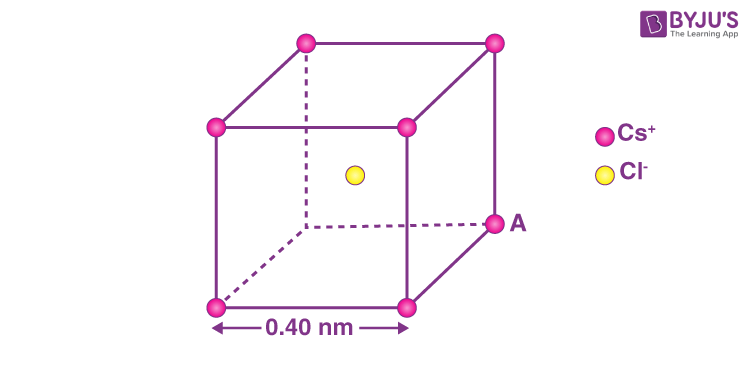• What is the net electric field on the Cl atom due to eight Cs atoms?
• Suppose that the Cs atom at corner A is missing. What is the net force now on the Cl atom due to the seven remaining Cs atoms?

Solution: (a) The chlorine atom at the cube’s centre is evenly attracted by eight cesium atoms at the cube’s eight corners. Symmetry points out that these forces cancel out in pairs. Thus, the total electric field on the Cl atom is zero.

$$\begin{array}{l} E = \frac{ F }{ q } \textup{ where } F = 0 \end{array}$$

$$\begin{array}{l} \therefore E = 0 \end{array}$$

(b) Since the Cs+ at point A is missing, then the total resultant on Cl ion will only be due to its opposite Cs+ ion, and the rest of the forces cancel out.

Total forces on Cl when Cs+ from point A is removed can be calculated using Coulomb’s law as follows:,

$$\begin{array}{l}F = \frac{ e ^2 }{ 4 \pi \varepsilon _ { 0 } r ^ { 2 } } \end{array}$$

r = distance between Cs ion and Cl ion,

$$\begin{array}{l} r = \sqrt{ ( 0.20 ) ^ { 2 } + ( 0.20 ) ^ { 2 } + ( 0.20 ) ^ { 2 } } \times 10 ^ { -9 } m \end{array}$$

$$\begin{array}{l} = 0.346 \times 10 ^ { -9 } m \end{array}$$

$$\begin{array}{l} F = \frac{ e ^ { 2 } }{ 4 \pi \varepsilon _ { 0 } r ^ { 2 } } \end{array}$$

$$\begin{array}{l} = \frac{ 9 \times 10 ^ { 9 } ( 1.6 \times 10 ^ { -19 } ) ^ { 2 } }{ ( 0.346 \times 10 ^ { -9 } ) ^ { 2 } } \end{array}$$

$$\begin{array}{l} = 1.92 \times 10 ^ { -9 } N \end{array}$$

4. Two charges -3q and q are placed fixed on the x-axis separated by distance ‘d’. Where should a third charge 2q be placed such that it will not experience any force?

Solution: Consider a charge 2q positioned at P, at a length l from point A, where charge q is positioned as displayed in the diagram.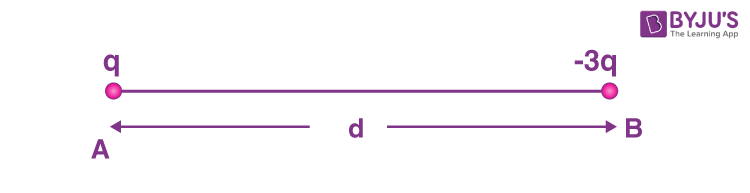The charge 2q does not experience any type of force when the repulsion force on it due to charge q is balanced by the attraction force on it due to -3q at B where AB = d.

$$\begin{array}{l}\textup{or } \frac{(2q)(q)}{4 \pi \varepsilon_0 l^2 } = \frac{(2q)(3q)}{4 \pi \varepsilon_0 (l + d)^2 }\end{array}$$

$$\begin{array}{l}(l + d)^2 = 3l^2 \textup{ or } 2l^2 – 2ld – d^2 = 0 \end{array}$$

Therefore,

$$\begin{array}{l}l = \frac{2d \pm \sqrt{4d^2 + 8d^2}}{4} + \frac{d}{2} \pm \frac{3d}{2} \Rightarrow l = \frac{d + \sqrt{3d}}{2} \end{array}$$

When charge 2q is positioned between B and A,

$$\begin{array}{l}l = \frac{( \sqrt{3} – 1 )}{2}d\end{array}$$

5. The below diagram shows the electric field lines around three point charges A, B, and C.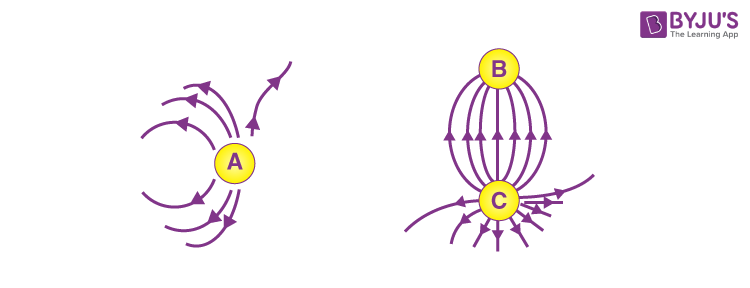• (a) Which charges are positive?
• (b) Which charge has the largest magnitude? Why?
• In which regions of the picture could the electric field be zero? Justify your answer.

(i) near A, (ii) near B, (iii) near C, (iv) nowhere

Solution: In the given diagram, it shows that the electric field lines envelop the three-point charge A, B, and C.
(a) The charges C and A are positive as force lines emanate from them.

(b) The charge C possesses the largest magnitude since the maximum field lines are related to it.
(c) The correct answer is (i) near A.
Reason: No neutral point exists between a negative and a positive charge. A neutral point may occur between the two like charges. From the given diagram, it is known that a neutral point occurs between charges C and A. In the case of between two like charges, the neutral point is nearer to the charge with a relatively lesser magnitude. So, the electric field has zero value near charge A.

6. Five charges, q each, are placed at the corners of a regular pentagon of side ‘a’.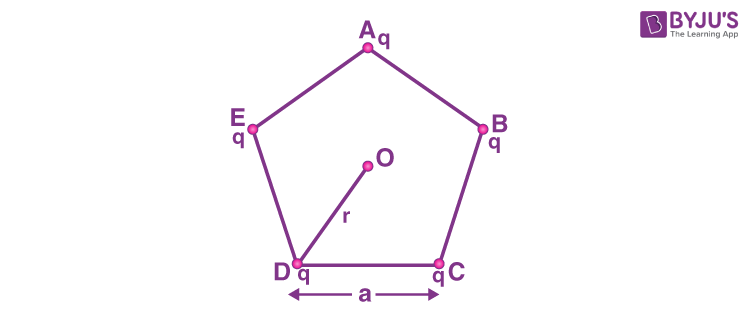• (a) What will be the electric field at 0, the centre of the pentagon?
• (b) What will be the electric field at 0 if the charge from one of the corners (say A) is removed?
• (c) What will be the electric field at 0 if the pentagon is replaced by an n-sided regular polygon with charge q at each of its corners?

Solution: (i) The point is O is placed equally from every charge at the endpoint of the pentagon. Therefore, because of symmetry, the forces due to every charge are cancelled out. As a consequence, the electric field is zero.

(ii) When charge q is taken away, a negative charge will arise at A lending electric field,

$$\begin{array}{l}E = \frac{q \times 1 }{4 \pi \varepsilon_0r^2 } \textup{along OA}\end{array}$$

(iii) If charge q is replaced by charge -q, then two negative charges -2q will be there. So, the value of the electric field is

$$\begin{array}{l}E = \frac{2q}{4 \pi \varepsilon_0 r^2} \textup{ along OA.}\end{array}$$

When the pentagon is replaced by an n-sided polygon with the charge at every corner, the electric field at point O continues to be zero as the charges’ symmetricity is due to the regularity of the polygon shape. In fact, it doesn’t rely on the number of sides or the charge numbers.

1. In 1959, Lyttleton and Bondi suggested that the expansion of the Universe could be explained if matter carried a net charge. Suppose that the Universe is made up of hydrogen atoms with a number density N, which is maintained as a constant. Let the charge on the proton be: ep = – (1 + y)e, where e is the electronic charge.

• (a) Find the critical value of y such that expansion may start.
• (b) Show that the velocity of expansion is proportional to the distance from the centre.

Solution: Let’s assume that our Universe is spherical in shape with a radius of R composed of H atoms.
The charge on the proton is equal to (1 + y)e

Therefore, the total number of charges on the H atom, eh = ep + e

= e + (1 + y)e

= e[-1 – y +1]

The charge on the 1H atom is equal to -ye.

$$\begin{array}{l}\textup{The number of H atoms in the spherical Universe} = N.\frac{4}{3} \pi R^3\end{array}$$

$$\begin{array}{l}\textup{Therefore, the net charge in the Universe} = N \frac{4}{3} \pi R^3 (-ye)\end{array}$$

Let’s assume the boundaries of the Universe as an ideal Gaussian surface. As per Gauss’s law of electrostatics,

$$\begin{array}{l}\oint_z E.ds = \frac{q}{\varepsilon_0} \end{array}$$

$$\begin{array}{l}E.4 \pi R^2 = \frac{-4 \pi N R^3 ye}{3 \varepsilon_0 }\end{array}$$

$$\begin{array}{l}E = \frac{-4 \pi NR^3ye}{3 \varepsilon_0 4 \pi R^2 } =\frac{-N R ye}{3 \varepsilon_0 }\end{array}$$

Coulomb force or electrostatic force acting on one H atom Fc = qE

$$\begin{array}{l}F_c = \frac{(-ye)(-NRye)}{3 \varepsilon_0 } \end{array}$$

$$\begin{array}{l}= \frac{+y^2e^2 NR}{3 \varepsilon_0 }\end{array}$$

Positive sign Fc displays repulsive force.

It is known that the gravitational potential at the Universe’s boundary is equal to GM/R2.

Mass of the Universe = M ( of all H number of atoms)

Therefore, the mass of the Universe (M) = Number of H atoms in the Universe × mp

$$\begin{array}{l}= N \frac{4}{3} \pi R^3m_p\end{array}$$

$$\begin{array}{l}F_G = \frac{G\left ( N\frac{4}{5} \pi R^3 \right )}{} m^2_p\end{array}$$

$$\begin{array}{l}F_G = \frac{G\left ( N\frac{4}{3} \pi R^3 \right )m^2_p}{R^2}\end{array}$$

$$\begin{array}{l}\Rightarrow F_G = \frac{4 \pi GNR m^2_p}{3} \end{array}$$

When FC > FG, the Universe will begin to expand. Therefore, the critical value of y’s expansion will be when FC = FG.

2. Consider a sphere of radius R with charge density distributed as

ρ (r) = kr for r ≤ R

= 0 for r> R

• (a) Find the electric field at all points r.
• (b) Suppose the total charge on the sphere is 2e, where e is the electron charge. Where can two protons be embedded such that the force on each of them is zero? Assume that the introduction of the proton does not alter the negative charge distribution.

Solution: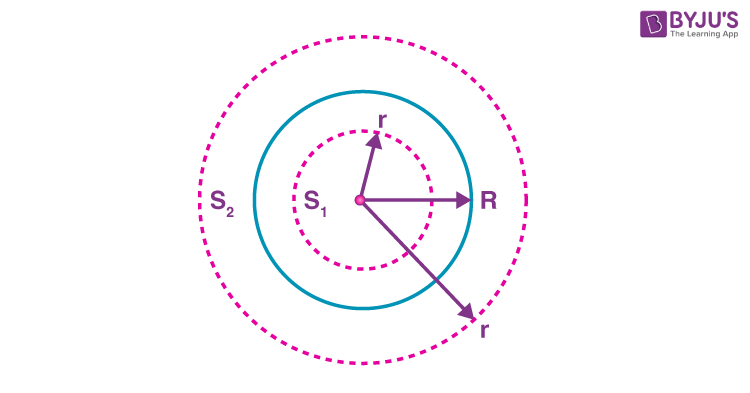Here, the charge density distribution of the given sphere is

$$\begin{array}{l}\rho(r) = kr \,\,\, r \leq R\end{array}$$

$$\begin{array}{l}\rho (r) = 0 \,\,\, r>R\end{array}$$

The electric field is the radius, as displayed in the diagram.

Let’s assume a spherical Gaussian surface S1 with radius r. Then on this surface,

$$\begin{array}{l}\oint \vec{E}. \vec{ds} = \frac{1}{ \varepsilon_0} \int \rho dV\end{array}$$

$$\begin{array}{l}\textup{Since } V = \frac{4}{3} \pi r^3, \end{array}$$

$$\begin{array}{l}r^2 dr = 4 \pi r^2dr \textup{ and } \rho (r) = kr \end{array}$$

$$\begin{array}{l}\therefore \oint \vec{E} . \vec{ds} = \frac{1}{ \varepsilon_0}4 \pi k \int_0^r r r^2 dr \end{array}$$

$$\begin{array}{l}(E) 4 \pi r^2 = \frac{4 \pi k }{ \varepsilon_0 } \frac{r^4}{4}\end{array}$$

$$\begin{array}{l}E = \frac{1}{4 \varepsilon_0 } kr^2 \textup{ …..(i)}\end{array}$$

$$\begin{array}{l}\textup{The direction } \vec{E} \textup{ is radially outwards (for positive charge density)}.\end{array}$$

$$\begin{array}{l}\textup{For points r } > \textup{R, let’s consider a spherical Gaussian surface } \textup{S}_2 \textup{ with radius r. Then on this surface, when r} = \textup{R}\end{array}$$

$$\begin{array}{l}\oint \vec{E}. \vec{ds} = \frac{1}{ \varepsilon_0 } \int \rho . dV \end{array}$$

$$\begin{array}{l}E (4 \pi r^2) = \frac{4 \pi k }{ \varepsilon_0} \int_0^R r^3 dr = \frac{4 \pi k }{ \varepsilon_0 } dr\end{array}$$

$$\begin{array}{l}= \frac{4 \pi k}{ \varepsilon_0 } \frac{R^4}{4}\end{array}$$

$$\begin{array}{l}E = \frac{k}{4 \varepsilon_0 } \frac{R^4}{r^2}\end{array}$$

$$\begin{array}{l}\textup{The direction of }\vec{E} \textup{ is radially outwards (in the case of positive charge density).}\end{array}$$

(b) From the principle of symmetry, it can be found that the two protons should be on the centre’s opposite sides, along the sphere’s diameter, as represented in the above diagram.
The charge on the sphere,

$$\begin{array}{l}q = \int_0^R \rho dV = \int_0^R (kr) 4 \pi r^2 dr \end{array}$$

$$\begin{array}{l}= 4 \pi k \frac{R^4}{4} =2e\end{array}$$

$$\begin{array}{l}\textup{Therefore, } k = \frac{2e}{ \pi R^4 } \textup{ …..(ii)}\end{array}$$

When protons 2 and 1 are attached at a distance r from the centre of the sphere, as represented in the diagram, the attractive force on proton 1 due to charge distribution is

$$\begin{array}{l}F_1 = -eE = -e \frac{kr^2}{4 \varepsilon_0 } \textup{ …..using (i)}\end{array}$$

The repulsive force acting on proton 1 due to proton 2 is

$$\begin{array}{l}F_2 = \frac{e^2}{4\pi \varepsilon_0 (2r)^2 }\end{array}$$

$$\begin{array}{l}\textup{Net force on proton 1 } F = F_1 + F_2 = – e \frac{kr^2}{4 \varepsilon_0 } + \frac{e^2}{16 \pi \varepsilon_0 r^2 }\end{array}$$

$$\begin{array}{l}F = \left [ – \frac{er^2}{4 \pi \varepsilon_0 } \frac{2e}{ \pi R^4 } + \frac{e^2}{16 \pi \varepsilon_0 r^4 } \right ]\end{array}$$

Here, the net force on proton 1 is zero,

$$\begin{array}{l}\textup{when } \frac{er^2.2e}{4 \varepsilon_0 } = \frac{e^2}{16 \pi \varepsilon_0 r^2 } \textup{ or } r^4 = \frac{R^4}{8} \textup{ or } r =\frac{R}{(8)^{\frac{1}{4}}}\end{array}$$

3. Two fixed, identical conducting plates (α & β), each of surface area S, are charged to -Q and q, respectively, where Q > q > 0. A third identical plate (γ), free to move, is located on the other side of the plate with charge q at a distance d (diagram). The third plate is released and collides with plate β. Assume the collision is elastic and the time of collision is sufficient to redistribute charge g amongst β & γ.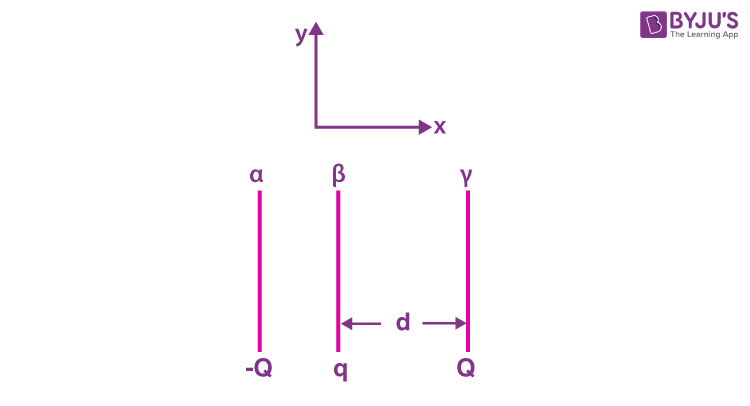• (a) Find the electric field acting on the plate γ before the collision.
• (b) Find the charges on β and γ after the collision.
• (c) Find the velocity of the plate γ after the collision and at a distance d from the plate β.

Solution: (a) The electric field around plate γ stimulated by the plate α is

$$\begin{array}{l}E_1 = – \frac{Q}{S(2 \varepsilon_0 )}, \textup{ to the left}\end{array}$$

The electric field around the plate γ stimulated by the plate β is

$$\begin{array}{l}E_2 = \frac{q}{S(2 \varepsilon_0 )}, \textup{to the right}\end{array}$$

Therefore, the total electric field at plate γ before the collision is

$$\begin{array}{l}E = E_1 + E_2 = \frac{q-Q}{S(2 \varepsilon_0 )}, \textup{ to the left if Q} > \textup{ q}\end{array}$$

(b) In the course of a collision, plate γ and β together. Both potentials become the same. Let’s assume that the charge on plane βisq1 and the charge on the plate γisq2. At any point O, in between the two plates, diagram, the electric field must be zero.

The electric field at 0 due to the plate

$$\begin{array}{l}\alpha = \frac{(-)Q}{S(2 \varepsilon_0 )}, \textup{to the left}\end{array}$$

The electric field at 0 due to the plate

$$\begin{array}{l}\beta = \frac{q_1}{S(2 \varepsilon_0 )}, \textup{to the right} \end{array}$$

The electric field at 0 due to the plate

$$\begin{array}{l}\gamma = \frac{q_2}{S(2 \varepsilon_0 )}, \textup{to the left}\end{array}$$

Since the electric field at 0 is zero, so,

$$\begin{array}{l}\frac{Q + q_2}{S(2 \varepsilon_0 )} = \frac{q_1}{S(2 \varepsilon_0 )}\end{array}$$

$$\begin{array}{l}\textup{Therefore } Q + q_2 = q_1 \textup{ or } Q = q_1 – q_2 \textup{ …..(i)}\end{array}$$

$$\begin{array}{l}\textup{On solving (i) and (ii), we get }q_1 = (Q + \frac{q}{2}) = \textup{charge on plate } \gamma \end{array}$$

(c) After the collision, at a length d from the plate β, assume that the velocity of plate γ is v.

The electric field at plate γ is

$$\begin{array}{l}E_2 = \frac{ -Q }{2 \varepsilon_0 S } + \frac{(Q + \frac{q}{2})}{2 \varepsilon_0 S}\end{array}$$

$$\begin{array}{l}= \frac{ \frac{q}{2} }{2 \varepsilon_0 S } \textup{ to the right}\end{array}$$

Just before the collision, the electric field around plate γ is

$$\begin{array}{l}E_1 = \frac{Q – q }{2 \varepsilon_0 S }\end{array}$$

If F1 is the force on plate γ before the collision, then,

$$\begin{array}{l}F_1 = E_1 Q = \frac{(Q – q)Q }{2 \varepsilon_0 S }\end{array}$$

Likewise, F2 on the plate γ after the collision,

$$\begin{array}{l}F_2 = E_2 \frac{q}{2} = \frac{(\frac{q}{2})^2}{2 \varepsilon_0 S}\end{array}$$

Net work done by the electric field in round trip motion of the plate γ

$$\begin{array}{l}W = (F_1 + F_2) d = \frac{[(Q-q)Q + (q/2)^2]d}{2 \varepsilon_0 S } \end{array}$$

If m is the mass of plate γ, the KE gained by the plate is

$$\begin{array}{l}\gamma = \frac{1}{2} mv^2\end{array}$$

As per the work done principle,

$$\begin{array}{l}\frac{1}{2} mv^2 = W = \frac{(Q -q/2 )^2 d}{2 \varepsilon_0 S }\end{array}$$

$$\begin{array}{l}v = (Q – q/2) \left ( \frac{d}{m \varepsilon_0 s } \right )^{ 1/2 }\end{array}$$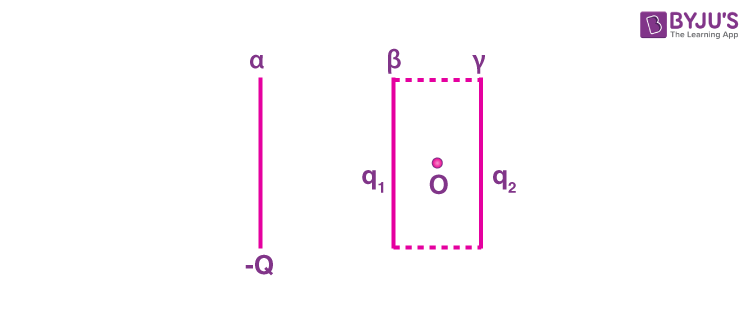4. There is another useful system of units besides the SI/mks. A system called the cgs (centimetre-gram-second) system. In this system, Coulomb’s law is given by

$$\begin{array}{l}F = \frac{Qq}{r^2} \hat{r}\end{array}$$

Where the distance r is measured in cm ( = 10-2 m), F in dynes ( = 10-5 N) and the charges in electrostatic units (es units), where 1es unit of charge = 1/|3| * 10-9 C

The number  actually arises from the speed of light in the vacuum, which is now taken to be exactly given by c = 2.99792458 × 108 m/s. An approximate value of c then is c =  × 108 m/s.

(i) Show that Coulomb’s law in cgs units yields
1 esu of charge = 1 (dyne) 1/2 cm

Obtain the dimensions of units of charge in terms of mass M, length L and time T. Show that it is given in terms of fractional powers of M and L.
(ii) Write 1 esu of charge = x C, where x is a dimensionless number. Show that this gives

$$\begin{array}{l}\frac{1}{4 \pi \varepsilon_0 } = \frac{10^{-9}}{x^2} \frac{N.m^2}{C^2}\end{array}$$

$$\begin{array}{l}\textup{With } x = \frac{1}{} \times 10^{-9}, \textup{we have}\end{array}$$

$$\begin{array}{l}\frac{1}{4 \pi \varepsilon_0 } = ^2 \times 10^9 \frac{Nm^2}{C^2}\end{array}$$

$$\begin{array}{l}\textup{or, } \frac{1}{4 \pi \varepsilon_0 } = (2.99792458)^2 \times 10^9 \frac{Nm^2}{C^2} (\textup{exactly}) \end{array}$$

Solution:

(I)

$$\begin{array}{l}F =\frac{Q_q}{r^2} = 1 \,\, dyne\end{array}$$

$$\begin{array}{l}= \frac{[1esu \,\, of \,\, charge]^2}{[1cm]^2}\end{array}$$

Therefore,

$$\begin{array}{l}[1esu \,\, of \,\, charge] = [F]^{\frac{1}{2L}}\end{array}$$

$$\begin{array}{l}= [MLT^{-2}]^\frac{1}{2L} \end{array}$$

$$\begin{array}{l}= M^{\frac{1}{2L}\frac{3}{2}}T^{-1}\end{array}$$

$$\begin{array}{l}[1esu \,\, of \,\, charge] = M^{\frac{1}{2L}\frac{3}{2}}T^{-1}\end{array}$$

Therefore, charge in the cgs unit is represented as fractional powers (½) of M and (3/2) of l.

(ii) Let’s assume the coulomb force on the two charges, each magnitude of 1 esu of charge separated by 1cm;

Then, the force of 1 dyne is equal to 10-5 N.

This scenario is equal to two charges of magnitude xC divided by 10-2 m.

This implies,

$$\begin{array}{l}F = \frac{1}{4 \pi \varepsilon_0 }. \frac{x^2}{10^{-4}} \textup{ which should be 1 dyne = }10^{-5} N\end{array}$$

Therefore,

$$\begin{array}{l}\frac{1}{4 \pi \varepsilon_0 }.\frac{x^2}{10^{-4}} = 10^{-5} \end{array}$$

$$\begin{array}{l}\frac{1}{4\pi \varepsilon_0 } = \frac{10^{-9}Nm^2}{x^2 C^2}\end{array}$$

$$\begin{array}{l}\textup{With x = } \frac{1}{} \times 10^9\end{array}$$

These results,

$$\begin{array}{l}\frac{1}{4 \pi \varepsilon_0 } = 10^{-9} \times ^2 \times 10^{18}\end{array}$$

$$\begin{array}{l}= ^2 \times 10^9 Nm^2 / C^2\end{array}$$

$$\begin{array}{l}\textup{With } \to 2.99792458, \end{array}$$

$$\begin{array}{l}\frac{1}{4 \pi \varepsilon_0 } = 8.98755 … \times 10^9 \frac{Nm^2}{C^2} \end{array}$$

5.Two charges -q each is fixed and separated by distance 2d. A third charge q of mass m placed at the mid-point is displaced slightly by x (x<<d) perpendicular to the line joining the two fixed charges, as represented in the figure. Show that q will perform simple harmonic -q oscillation of time period.

$$\begin{array}{l}T = \left [ \frac{8 \pi^2 \varepsilon_0 md^3]^{1/2} }{q^2} \right ]\end{array}$$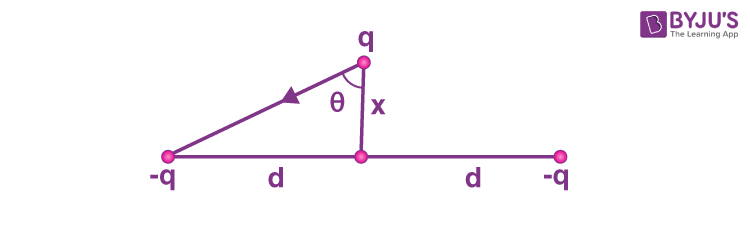Solution:

Force on charge q due to -q charge at A is FPA, along P to A.
Therefore,

$$\begin{array}{l}FPA = \frac{1}{4 \pi \varepsilon_0 } \frac{q_1q_2}{r^2} = \frac{1}{4 \pi \varepsilon_0 } \frac{q(-q)}{r^2}\end{array}$$

$$\begin{array}{l}F_{PA} = \frac{-q^2}{4 \pi \varepsilon_0 r^2 }\end{array}$$

Likewise,

$$\begin{array}{l}F_{PB} = \frac{-q^2}{4 \pi \varepsilon_0 r^2 } [\because |F_{PA}| = |F_{PB}| = F]\end{array}$$

Therefore, the horizontal components of FPB and FPA are downward.

Total force on q at P = FPAcosθ + FPBcosθ = 2Fcosθ [∵|FPA| = | FPB | = F]

$$\begin{array}{l}= \frac{-2q^2}{4 \pi \varepsilon_0 } cos \theta\end{array}$$

$$\begin{array}{l}\textup{Therefore, force on q downwards} = \frac{-2q^2}{4 \pi \varepsilon_0 } \frac{x}{r}\end{array}$$

$$\begin{array}{l}r^2 = x^2 + d^2\,\,[\textup{By Pythagoras Theorem}]\end{array}$$

$$\begin{array}{l}\therefore \textup{Force on q} = \frac{-2q^2}{4 \pi \varepsilon_0 (x^2 + d^2)^{3/2}} \end{array}$$

$$\begin{array}{l}\because x <<< d \therefore x^2 <<<< d^2 \end{array}$$

The negative sign represents the force of attraction.

$$\begin{array}{l}\textup{Thus, the total force on charge q at P downwards } = \left ( \frac{-2q^2}{4 \pi \varepsilon_0 d^3 } \right ) x\end{array}$$

Therefore, the force on charge q is directly proportional to the displacement from mean location O (the midpoint of the segment joining -q and q charges). Thus, the movement of q about will be SHM.

$$\begin{array}{l}F = -kx, \textup{where, } k = \frac{2q^2}{4\pi \varepsilon_0d^3 }\end{array}$$

Therefore,

$$\begin{array}{l}\omega = \sqrt{ \frac{k}{m} }\end{array}$$

$$\begin{array}{l}T = \frac{2 \pi }{ \omega } = 2 \pi \sqrt{ \frac{m}{k} } \end{array}$$

$$\begin{array}{l}T = 2 \pi \sqrt{ \frac{ \frac{m}{2q^2} }{ 4 \pi \varepsilon_0d^3 } } = 2 \pi \sqrt{ \frac{ 4 \pi m \varepsilon_0 d^3 }{ 2q^2 } }\end{array}$$

$$\begin{array}{l}T = \left [ \frac{ 8 \pi ^3 \varepsilon_0 md^3 }{q^2} \right ]^{ \frac{1}{2} } \end{array}$$

Hence proved.

6.Total charge -Q is uniformly spread along the length of a ring of radius R. A small test charge +q of mass m is kept at the centre of the ring and is given a gentle push along the axis of the ring.

• (a) Show that the particle executes a simple harmonic oscillation.
• (b) Obtain its time period.

Solution:

(a) Allow the charge q to be displaced towards the axis of the ring.

The electric field around the axis of the ring at a length z from the axis is

$$\begin{array}{l}E = \frac{1}{ 4\pi \varepsilon_0 } \frac{Qz}{ (R^2 + Z^2)^{3/2} }\end{array}$$

Fnet = qE

$$\begin{array}{l}F_{net} = \frac{1}{ 4 \pi \varepsilon_0 } \frac{qQz}{(R^2 + Z^2 )^{3/2}} \end{array}$$

$$\begin{array}{l}\because z \ll R \end{array}$$

$$\begin{array}{l}F_{net} = \frac{1}{4 \pi \varepsilon_0 } \frac{qQz}{R^3}\end{array}$$

$$\begin{array}{l}\textup{Let, } K = \frac{1}{4 \pi \varepsilon_0 } \frac{qQ}{R^3}\end{array}$$

$$\begin{array}{l}\textup{So, } F_{net} = Kz\end{array}$$

The force is directly proportional to the z and pointed towards the point O. The total force can be expressed as

Fnet = -Kz

This conveys that the particles perform SHM.

(b) We know that

$$\begin{array}{l}\omega = \sqrt{ \frac{K}{m} }\end{array}$$

$$\begin{array}{l}T = \frac{2\pi}{\omega }\end{array}$$

$$\begin{array}{l}T = 2 \pi \sqrt{ \frac{m}{K} }\end{array}$$

$$\begin{array}{l}T = 2 \pi \sqrt{ \frac{ m }{ \frac{1}{ 4 \pi \varepsilon_0 } \frac{pQ}{R^3} } }\end{array}$$

$$\begin{array}{l}T = 2 \pi \sqrt{ \frac{ 4 \pi \varepsilon_0 mR^3 }{Qq} }\end{array}$$

## Watch the video on Electric Charges and Fields problems frequently asked in NEET Exam